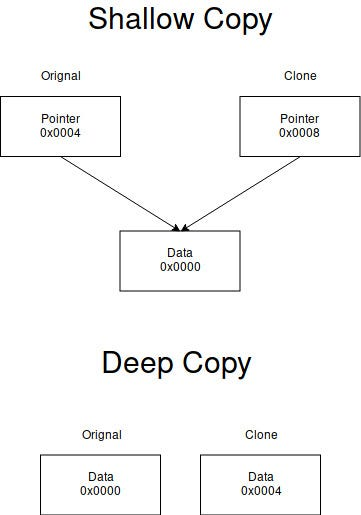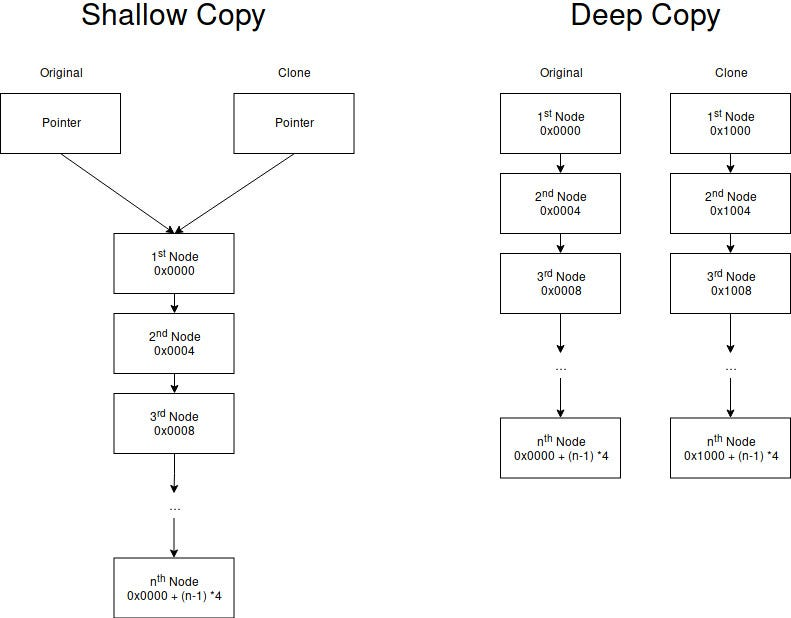# PHP 的複合類型（compound type）

### 實驗

`\$arr = ['a' => 1];\$obj = (object) ['a' => 1];\$callable = function () { return 1; };`
`function changeArray(array \$a) {    \$a['a'] = 2;}`
`function changeObject(object \$o) {    \$o->a = 2;}`
`function changeCallable(callable \$c) {    \$c = function () { return 2; };}`

`changeArray(\$arr);`
`changeObject(\$obj);`
`changeCallable(\$callable);`

### 說明

#### 淺拷貝（Shallow Copy）與深拷貝（Deep Copy）

1. 申請一個記憶體位置，並儲存欲拷貝的值所在的記憶體位置。
此複製方式稱為淺拷貝。
2. 申請一個記憶體位置，將欲拷貝的值重新在這個記憶體位置中儲存。
此複製方式稱為深拷貝。在 Single Object下的 Shallow Copy 及 Deep Copy在 Linked List 下的 Shallow Copy 及 Deep Copy

Shallow Copy 的空間複雜度為 `O(1)`時間複雜度為 `O(1)`
Deep Copy 的空間複雜度為 `O(n)`時間複雜度為 `O(n)`

#### 設計理念？

`let x = 5;let y = x;`
`// 在此處，x 及 y 都是可以合法使用的變數println!("x = {}, y = {}", x, y);`

`let s1 = String::from("hello");let s2 = s1;`
`// 在此處，s1 已不再是合法可以使用的變數，僅能使用 s2// 因為 s1 的指標已被 Move 至 s2，而 Rust 預設是不允許無效的指標引用`
`println!("{}, world", s2);`

`array`因為它在被建立的時候就知道其記憶體大小（更新時也一併會更新該值），故設計上可以採用 Deep Copy。

#### 如何繞過

`function changeArray(array &\$arr) {    \$arr['a'] = 2;}`
`function changeCallable(callable &\$callable) {    \$callable = function () { return 2; };}`

`function changeObject(object \$object) {    \$newObj = clone \$object;    \$newObj->a = 2;}`